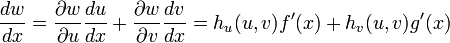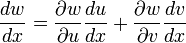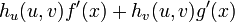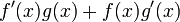# Proof of product rule for differentiation using chain rule for partial differentiation

This article proves the product rule for differentiation in terms of the chain rule for partial differentiation.

## Statements

### Statement of product rule for differentiation (that we want to prove)

uppose$f$ and$g$ are functions of one variable. Then the following is true wherever the right side expression makes sense (see concept of equality conditional to existence of one side):$\! \frac{d}{dx}[f(x)g(x)] = f'(x)g(x) + f(x)g'(x)$

### Statement of chain rule for partial differentiation (that we want to use)

Suppose$f,g$ are both functions of one variable and$h$ is a function of two variables. Suppose$u = f(x), v = g(x), w = h(u,v)$. Then:$\frac{dw}{dx} = \frac{\partial w}{\partial u}\frac{du}{dx} + \frac{\partial w}{\partial v} \frac{dv}{dx} = h_u(u,v)f'(x) + h_v(u,v)g'(x)$

## Proof

Given: Functions$f$ and$g$

To prove:$\! \frac{d}{dx}[f(x)g(x)] = f'(x)g(x) + f(x)g'(x)$ wherever the right side makes sense.

Proof:

Consider the function:$\! h(u,v) := uv$

Its partial derivatives are:$\! h_u(u,v) = v, \qquad h_v(u,v) = u$ Define:$\! u := f(x), \qquad v := g(x), \qquad w := uv = h(u,v) = f(x)g(x)$

By the chain rule for partial differentiation, we have:$\! \frac{dw}{dx} = \frac{\partial w}{\partial u} \frac{du}{dx} + \frac{\partial w}{\partial v}\frac{dv}{dx}$

The left side is$(fg)'(x)$. The right side becomes:$\! h_u(u,v)f'(x) + h_v(u,v)g'(x)$

This simplifies to:$\! vf'(x) + ug'(x)$

Plug back the expressions$u = f(x), v = g(x)$ and get:$\! f'(x)g(x) + f(x)g'(x)$